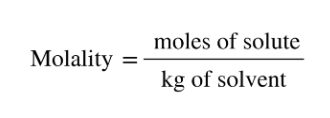# Problem: What is the molality of phosphoric acid, H3PO4, in a solution of 14.5 g of H3PO4 in 125 g of water?(a) Outline the steps necessary to answer the question.(b) Answer the question.

###### FREE Expert Solution
• Recall that molality (m) can be calculated as• Start by calculating the moles of H3PO(solute) using the mass provided and the molar mass
• Convert the mass of solvent (water) from g to kg
96% (481 ratings)###### Problem Details

What is the molality of phosphoric acid, H3PO4, in a solution of 14.5 g of H3PO4 in 125 g of water?

(a) Outline the steps necessary to answer the question.